# SAT Math Multiple Choice Question 417: Answer and Explanation

### Test Information

Question: 417

12.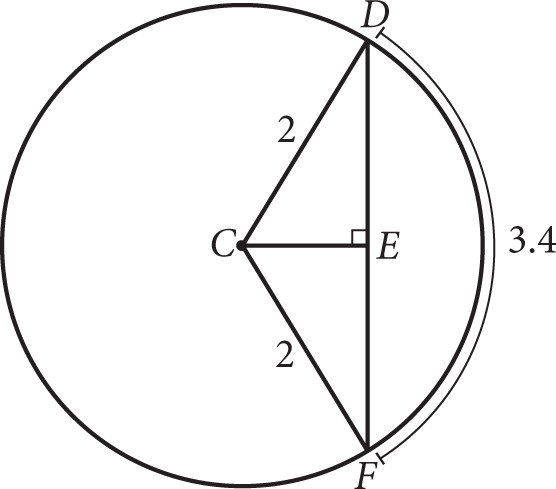Which of the following gives the length of chord DF in the figure above?

• A. 2cos(1.7)
• B. 2sin(1.7)
• C. 4cos(0.85)
• D. 4sin(0.85)

Explanation:

D

Difficulty: Hard

Category: Additional Topics in Math / Trigonometry

Strategic Advice: This is a very difficult question involving arc length given in radians and answer choices that involve trig functions. If you're not familiar with these topics, you should guess and move on to the next question. (Don't forget—there is no penalty for wrong answers on the new SAT!)

Getting to the Answer: Take a peek at the answer choices—the angles of the trig functions are given in radians, rather than degrees (you know this because there is no degree symbol). This means you'll need to use the radian formula for finding arc length: arcL = θ × r, where θ is the central angle of the arc in radians and r is the radius of the circle. This will allow you to determine the measure of the central angle, half of which becomes one of the angles of a right triangle (CDE, for example). You know both the arc length (3.4) and the radius (2), so solve for the central angle.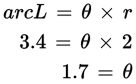This means that ∠DCF has a measure of 1.7 radians, and consequently, ∠DCE has a measure of half that, or 0.85 radians. Add this measure to the triangle, or draw a quick right triangle off to the side like the one below: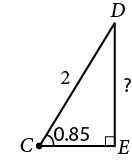Now, if you can find the length of side DE, you can double it to find the length of chord DF. Side DE is opposite the angle measure that you found and you know the hypotenuse of the triangle; so, use the ratio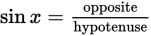to find the length of side DE: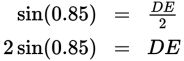Multiply by 2 to find that DF = 2 × 2sin(0.85) = 4sin(0.85). Keep in mind that multiplying the angle (inside the parentheses) is not the same as multiplying the whole quantity by 2.